Kotlin: An Illustrated Guide • Chapter 4

# Introduction to Classes and Objects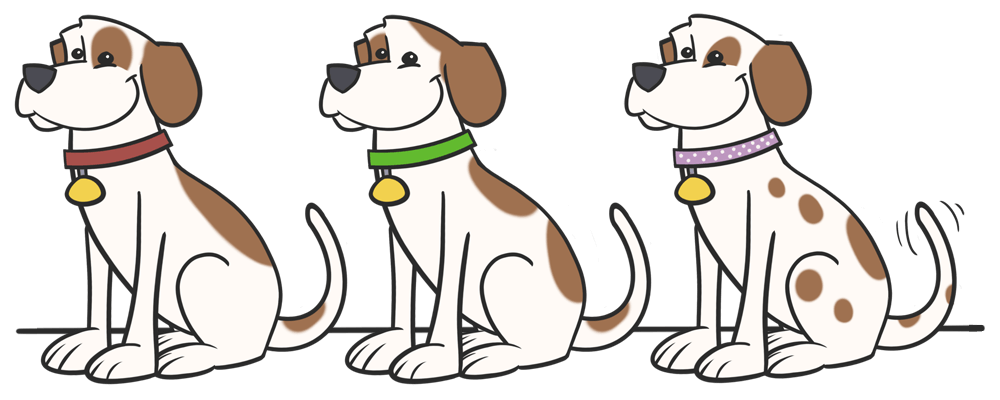Way back in chapter 1, we learned about a variety of types in Kotlin, such as `Double`, `String`, and `Boolean`. In this chapter, we’re going to start creating our very own types.

## Putting Variables and Functions Together

As you might recall, we started off this series creating variables to hold things pertaining to a circle, such as its radius and circumference. And in chapter 2, we created a function to calculate the circumference from the radius.

It all looked kind of scrambled, like this:This was manageable for such a simple example, but as we come up with more and more things that we want to know about the circle, such as its diameter, area, or position, it can become quite difficult to manage all of those different variables and functions. And once we start introducing other shapes, like rectangles and triangles, it becomes even harder to keep things straight - for example, which functions give us the area of a circle, and which give us the area of a rectangle?

So in this chapter, we’ll create a new type called a `Circle`. This way, instead of having separate variables and functions that hold a radius and a circumference, we can have a single variable that represents the circle itself.

So instead of the scrambled variables and functions above, it’ll look more like this:In Kotlin, we can put related variables and functions together using a class, which is a feature that we will use to create our new `Circle` type.

## Defining a Class

Let’s create our very first class, a `Circle`, which will include these variables and functions:

• The `radius` variable
• The read-only `pi` variable
• The `circumference()` function

Instead of jumping into all of this at once, let’s build up this class slowly… one step at a time… and look carefully at each part.

### An Empty Class

First, let’s define an empty class - with no variables and no functions.

``class Circle``

This is all it takes to create a new class called `Circle`!

When we create a class, we’re creating a new type, just like `Int`, `Double`, and `String`. In other words, once we define the `Circle` class like this, we can use it anywhere that we’d normally put a type, such as a function parameter.

``````fun draw(circle: Circle) {
// Code that draws the circle would go here
}``````

### Our First Diagram

It’s often useful to diagram your classes, so that you can visualize them and communicate your ideas to your friends. We’ll also use diagrams throughout the rest of this course to help explain concepts.

So, as we’re building out this `Circle` class, we’ll also diagram it at each stage, using a diagramming standard called the Unified Modeling Language, or UML for short.

We’ll start simple. To show a class, just put the name of the class in a box, like this. (I’ll also add the Kotlin code alongside the diagram, so that you can compare them).``````class Circle ``````

Now that we’ve got our new `Circle` type, we could create a variable to hold it, but since our class is completely empty, it’s not particularly useful yet. So, before we do that, let’s give our `Circle` a `radius`!

### Adding a `radius` Property

In real life, every circle has a radius, so we need to make sure that every `Circle` in our code also has a `radius` variable. Here’s how we can do that:

``class Circle(var radius: Double)``

Here, we added a new variable to the class, called `radius`, which has a type of `Double`. The value of `radius` can be changed, because of the `var` that comes before it. In Kotlin, a variable in a class like this is called a property of the class.

Let’s also update our diagram to show that a `Circle` class has a `radius` property. To do this, we draw a horizontal line under the name of the class, and write out the name of the property and its type, almost identically to how we write it in our Kotlin code:``````class Circle(var radius: Double) ``````

Now that we’ve got a circle class with a radius, we’re ready to start using it!

## Objects

In this series, we’ve already created lots of variables. For example, here’s how we can declare and assign a variable with a `Double` type:

``val radiusOfSmallCircle: Double = 5.2``

As mentioned, when we created the class, we made a new type called `Circle`. Just like you can have a variable that’s a `Double` type, you can also have a variable that’s a `Circle` type. Declaring and assigning a `Circle` variable is easy:

``val smallCircle = Circle(5.2)``

This creates a new variable called `smallCircle`, which is assigned a `Circle` with a radius of `5.2`.

Keep in mind - just as you can have many different `Double` values like…

• `5.2`
• `6.7`
• `10.0`

… you can also have lots of different `Circle` values like…

• A circle with a radius of `5.2`
• A circle with a radius of `6.7`
• A circle with a radius of `10.0`

But when it comes to classes, instead of calling these values, we usually call them objects.

### Constructing Objects

Let’s look at that code again.

``````// Declaring the class

// Using the class
val smallCircle = Circle(5.2)
``````

What’s happening when we write `Circle(5.2)`?

It’s kind of like we’re calling a function named `Circle()` that has a parameter called `radius` and a return type of `Circle`. These kinds of functions aren’t called functions, though. They’re called constructors because they construct a new object.

#### Constructor Parameters

Note that when you call a constructor, you must provide an argument for every property that is listed between the constructor’s opening and closing parentheses - `(` and `)`. Since we put `var radius: Double` between those parentheses, we have to provide an argument of type `Double` when we call the constructor: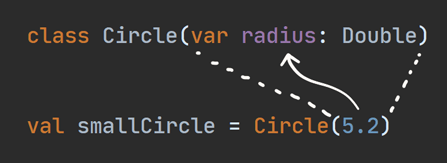Note that `radius` is actually filling two roles:

1. It’s a constructor parameter. You have to provide a value for it whenever you call the constructor. (However, just like with function parameters, you can also give a constructor parameter a default argument).
2. It’s a property. You’ll be able to get the value of the `radius` from any circle object. We’ll see an example of this in a moment.

When you create an object, we say that you’re creating an instance of the class. For that reason, creating an object is sometimes referred to as instantiating the class.

### Classes vs. Objects

The difference between classes and objects can be confusing at first, so let’s take a moment to clarify it.

A class describes the characteristics and behavior of some concept. If we’re talking about circles, those characteristics might include its radius, diameter, circumference, and area.

An object is an actual particular instance of that thing. Here are three circle objects: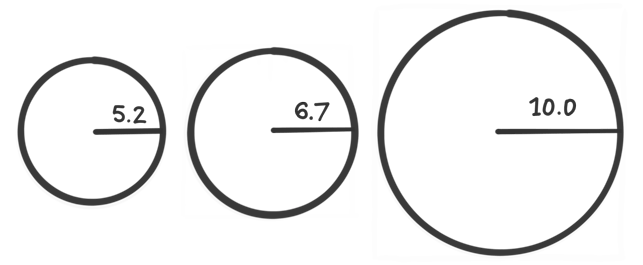A circle class answers questions like:

• “What does it mean for something to be a circle?”
• “What characteristics does it have?”
• “What does it do?”

A circle object answers questions like:

• “What is the radius of this particular circle?”
• “What is its circumference?”
• “What is its area?”

Here are a few more examples to help distinguish between classes and objects.

• You might have a Number class, with objects like 32,768 or 6.62607015.
• You might have a Color class, with objects like red, green, and blue.
• You might have a Dog class, with objects like Fido, Rover, or Mrs. Wagglytails.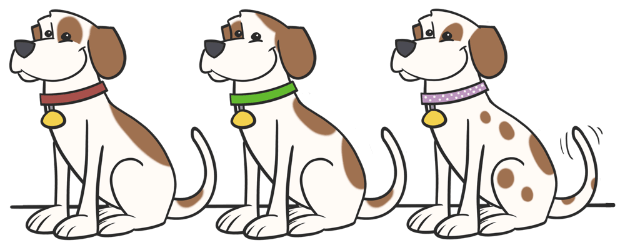We’ll see plenty more examples of classes and objects throughout the rest of this series!

### Getting a Property’s Value

Now that we’ve created a circle object, how can we get its radius?

Easy: to get the value of a property on an object, type the name of the variable, a dot `.`, and the name of the property, like this:

``````val smallCircle = Circle(5.2)

After running that code, `radiusOfSmallCircle` will be equal to `5.2`.

That takes care of `radius`. It’s time to move onto the other property, `pi`.

### Constant Properties

We could put `pi` inside the parentheses like we did for `radius`, separating them with a comma:

``class Circle(var radius: Double, val pi: Double)``

But if we do this, then we’d have to provide `pi` every time we instantiate a circle, like this:

``````val smallCircle = Circle(5.2, 3.14)
val mediumCircle = Circle(6.7, 3.14)
val largeCircle = Circle(10.0, 3.14)``````

Hmm… that’s not quite what we want. Since `pi` should always be the exact same value regardless of the particular circle, it doesn’t make sense for it to be a constructor parameter.

In fact, it would be better if the calling code could never specify the value of `pi` when constructing a `Circle`.

To do that, we can simply move `pi` out of the parentheses, so that it looks like this:

``````class Circle(var radius: Double) {
val pi: Double = 3.14
}``````

Here, we added an opening brace `{` and a closing brace `}`. Everything between those braces is called the body of the class. Inside of the body, we declare `pi`, and assign it a value of `3.14`.

By moving it out of the parentheses, the `pi` property is no longer a constructor parameter, so we can continue to call the constructor with just the radius, as we did back in Listing 4.5:

``val smallCircle = Circle(5.2)``

### Private Properties

As it is now, you can take any circle and get the value of both `radius` and `pi`:

``````val smallCircle = Circle(5.2)

val piFromSmallCircle = smallCircle.pi``````

I can’t think of too many reasons why code outside of the class would need the value of `pi`. Let’s make it so that this property is only visible from inside the class. To do that, we can add the keyword `private` when we declare it.

``````class Circle(var radius: Double) {
private val pi: Double = 3.14
}``````

Now, if you try to get the value of the `pi` property from outside of the class body, you’ll get an error:

``````val smallCircle = Circle(5.2)

val piFromSmallCircle = smallCircle.pi``````

Let’s update our UML class diagram to include the `pi` property. We can indicate the visibility of a property in the diagram:

• Private properties are preceded with a `-` symbol
• Public properties are preceded with a `+` symbol``````class Circle(var radius: Double) { private val pi: Double = 3.14 } ``````

Now, we’re ready to add the `circumference()` function to the class!

When a function belongs to a class, it’s often called a method or member function. Adding a method to a class is easy - just put it into the body of the class. To start with, let’s just take the exact same function from Listing 2.3, and plop it verbatim into our `Circle` class:

``````class Circle(var radius: Double) {
private val pi: Double = 3.14

}``````

When first we created the `circumference()` function back in Chapter 2, it made sense for it to have a parameter called `radius`. But now that we’re adding this function to the class, we can just refer to the value of the `radius` property instead.

In other words, instead of referring to the `radius` parameter, like this…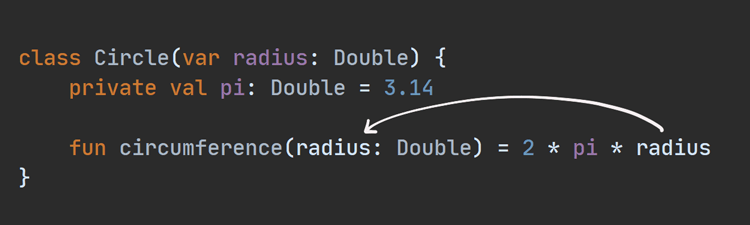… we can remove the `radius` parameter from the function, so that it refers to the property, like this…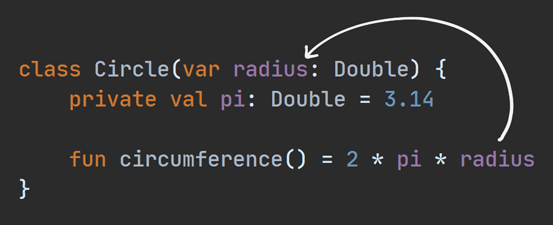This introduces the concept of scope, which we will explore in depth in an upcoming chapter. For now, just be sure to remove the parameter from the `circumference()` function so that `2 * pi * radius` will refer to the `radius` property.

``````class Circle(var radius: Double) {
private val pi: Double = 3.14

fun circumference() = 2 * pi * radius
}``````

Now that `Circle` has a `circumference()` function, how can we call it?

Calling a function on an object is done similarly to how we got the value of `radius`: the name of the variable, a dot `.`, and the name of the function.

``````val smallCircle = Circle(5.2)
val circumferenceOfSmallCircle: Double = smallCircle.circumference()``````

And before we move on, let’s add `circumference()` to our diagram!``````class Circle(var radius: Double) { private val pi: Double = 3.14 fun circumference() = 2 * pi * radius } ``````

Note that the diagram does not include the body of the function. In other words, `2 * pi * radius` does not appear in it. That’s because class diagrams are designed to give you an idea of what data and behavior are a part of the class, without going into the specifics.

When we started off this chapter, we only had a `radius` variable and a `circumference()` function. Now that we’ve put those two things together into a class, it’s time to fill out our `Circle` class with other things that you might want to know about a circle.

For example, we can easily add a function that calculates the area of the circle.

``````class Circle(var radius: Double) {
private val pi: Double = 3.14

fun circumference() = 2 * pi * radius
}

val smallCircle = Circle(5.2)
val areaOfSmallCircle = smallCircle.area()``````

We can also add a function to calculate its diameter.

``````class Circle(var radius: Double) {
private val pi: Double = 3.14

fun circumference() = 2 * pi * radius
fun diameter() = 2 * radius
}

val smallCircle = Circle(5.2)
val diameterOfSmallCircle = smallCircle.diameter()``````

We can even change how we calculate the circumference, by using the `diameter()` function instead:

``````class Circle(var radius: Double) {
private val pi: Double = 3.14

fun circumference() = diameter() * pi
fun diameter() = 2 * radius
}``````

And now, we can add these last few functions to our UML diagram.``````class Circle(var radius: Double) { private val pi: Double = 3.14 fun circumference() = diameter() * pi fun area() = pi * radius * radius fun diameter() = 2 * radius } ``````

## Anatomy of a Class

Now that we’ve covered the basics of Kotlin classes, here’s a recap of the main pieces: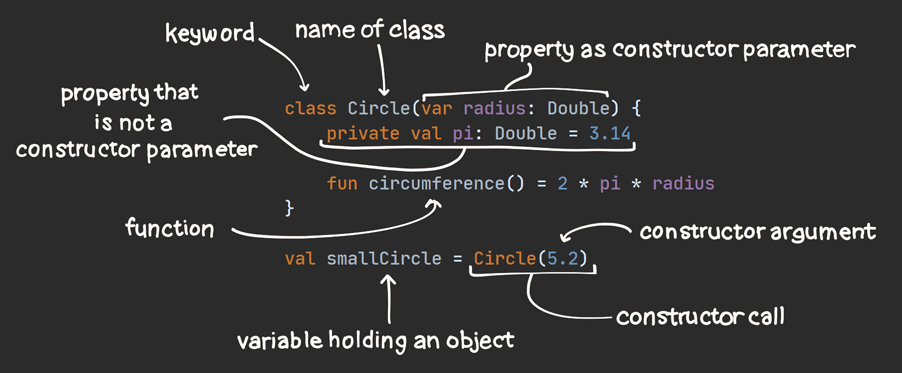## Everything is an Object

Up until this chapter, we’ve only used built-in Kotlin types, such as `Double`, `String`, and `Boolean`. You might be surprised to learn that when we used those types, we were actually using classes and objects! Just like we used the dot `.` to get properties and call functions on our `Circle` objects, we can also use a dot on any of these types.

### Doubles as Objects

For example, objects of type `Double` have functions like `plus()` and `times()`. So instead of writing our `circumference()` function like this…

``fun circumference() = 2 * pi * radius``

… we can instead write it like this …

``fun circumference() = 2.times(pi).times(radius)``

Kotlin developers normally use the arithmetic operators (`+`, `-`, `*`, `/`) in most cases, but the functions are there if you want them!

### Strings as Objects

`String` objects also have some interesting properties and functions. Here are a few examples:

• The `length` property tells you how many characters (i.e., letters, numbers, and symbols) are in the string.
• `toUpperCase()` will force all of the letters in the string to upper case.
• `drop()` will remove characters from the beginning of the string.

Here’s how that looks in code:

``````val greeting: String = "Welcome"

val numberOfLettersInGreeting = greeting.length // Evaluates to 7
val loudGreeting = greeting.toUpperCase()       // Evaluates to "WELCOME"
val substring = greeting.drop(3)                // Evaluates to just "come"``````

### Booleans as Objects

Even `Boolean` variables - which are only ever `true` or `false` - are objects! For example, if you want to turn on the headlights of your car if either it’s dark or it’s raining, you can write code to do that like this:

``````val isDark: Boolean = true
val isRaining: Boolean = false

val shouldStayHome = isDark.and(isRaining)``````

As you can see, objects are everywhere in Kotlin! Even simple values are objects.

## Summary

Classes are powerful, and even though we covered a lot of ground in this chapter, we’ve really only introduced them. They open up an entire new world of ways to represent your concepts in code. Later in this series, we’ll cover more advanced concepts like abstraction and inheritance. But for now, you should be proud of your progress!

In this chapter, we finally created our very own types, using classes. We learned:

• How to define a class
• How to create an object - that is, an instance of a class.
• How to add properties to a class, and how to access them.
• How to add functions to a class, and how to call them.
• How to create a UML diagram for a single class.
• How to call functions and get properties from the built-in Kotlin types that we’ve already worked with, such as `Double`, `String`, and `Boolean`.

And… just in case you haven’t had enough, you also can see a wide range of examples of classes in Kotlin.

In the next chapter, we’ll look at a special kind of class, called an enum class. See you then!

Thanks to Tobenna Ezike and Esraa Ibrahim for reviewing this chapter.